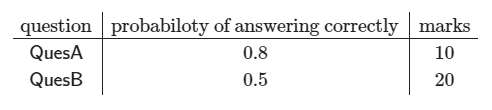Skip to content
Related Articles
GATE | GATE CS 2021 | Set 2 | Question 39
• Last Updated : 23 May, 2021

In an examination, a student can choose the order in which two questions (QuesA and QuesB) must be attempted.

• If the first question is answered wrong, the student gets zero marks.
• If the first question is answered correctly and the second question is not answered correctly, the student gets the marks only for the first question.
• If both the questions are answered correctly, the student gets the sum of the marks of the two questions.

The following table shows the probability of correctly answering a question and the marks of the question respectively.Assuming that the student always wants to maximize her expected marks in the examination, in which order should she attempt the questions and what is the expected marks for that order (assume that the questions are independent)?
(A) First QuesA and then QuesB. Expected marks 14
(B) First QuesB and then QuesA. Expected marks 14
(C) First QuesB and then QuesA. Expected marks 22
(D) First QuesA and then QuesB. Expected marks 16

Answer: (D)

Explanation:

There are 2 ways of solving this question-:

Method 1:
Expected marks from 1st Question = 0.8×10 = 8
Expected marks from 2nd Question = 0.8×0.5*20 = 8
(Now from the options you directly got D option)

Method 2:
If we want to focus on Order of questions then

First A then B

Expected Marks :

`0.8× 0.5×30 + 0.8×0.5×10 = 16 `

Explanation –
Probability of A is correct 0.8 and probability of B is correct is 0.5 when both are correct then we get 30 marks
Probability of A correct 0.8 and Probability of B is not correct is 0.5 then 0.8×0.5×10

First B then A

Expected Marks :

`0.5×0.8×30 + 0.5×0.2×20 = 14`

Explanation –
Probability of B is correct 0.5 and probability of A is correct is 0.8 when both are correct then we get 30 marks
Probability of B is correct 0.5 and probability of A is not correct is 0.2 so 0.5×0.2×20

Attention reader! Don’t stop learning now. Learn all GATE CS concepts with Free Live Classes on our youtube channel.

My Personal Notes arrow_drop_up